Definite Integrals MCQ - 1

# Definite Integrals MCQ - 1

Test Description

## 10 Questions MCQ Test Topic-wise Tests & Solved Examples for IIT JAM Mathematics | Definite Integrals MCQ - 1

Definite Integrals MCQ - 1 for Mathematics 2022 is part of Topic-wise Tests & Solved Examples for IIT JAM Mathematics preparation. The Definite Integrals MCQ - 1 questions and answers have been prepared according to the Mathematics exam syllabus.The Definite Integrals MCQ - 1 MCQs are made for Mathematics 2022 Exam. Find important definitions, questions, notes, meanings, examples, exercises, MCQs and online tests for Definite Integrals MCQ - 1 below.
Solutions of Definite Integrals MCQ - 1 questions in English are available as part of our Topic-wise Tests & Solved Examples for IIT JAM Mathematics for Mathematics & Definite Integrals MCQ - 1 solutions in Hindi for Topic-wise Tests & Solved Examples for IIT JAM Mathematics course. Download more important topics, notes, lectures and mock test series for Mathematics Exam by signing up for free. Attempt Definite Integrals MCQ - 1 | 10 questions in 30 minutes | Mock test for Mathematics preparation | Free important questions MCQ to study Topic-wise Tests & Solved Examples for IIT JAM Mathematics for Mathematics Exam | Download free PDF with solutions
 1 Crore+ students have signed up on EduRev. Have you?
Definite Integrals MCQ - 1 - Question 1

### Let f: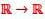be defined as f (t)  =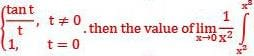f(t )dt

Definite Integrals MCQ - 1 - Question 2

### A minimum value of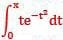is

Detailed Solution for Definite Integrals MCQ - 1 - Question 2

Correct Answer :- d

Explanation : f(x) = ∫(0 to x) te-t^2 dt

f'(x) = xe-x^2 = 0

Therefore, x = 0

f"(x) = e-x^2(1 - 2x2)

f"(0) = 1 > 0

Therefore, the minimum value is f(0) = 0

Definite Integrals MCQ - 1 - Question 3

### Let f(x) = ∫ex(x−1)(x−2)dx then f decrease in the interval

Detailed Solution for Definite Integrals MCQ - 1 - Question 3

f(x) = ∫ex(x−1)(x−2)dx

For decreasing function ,f′(x)<0,f′(x)<0

⇒ex(x−1)(x−2) < 0

⇒(x−1)(x−2) < 0

⇒ ex(x−1)(x−2) < 0

⇒ (x−1)(x−2) < 0
⇒1 < x < 2,

∵ e> 0 ∀ x ∈ R

Definite Integrals MCQ - 1 - Question 4

Evaluate: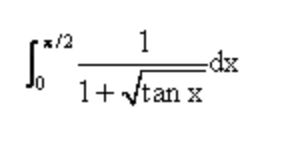Detailed Solution for Definite Integrals MCQ - 1 - Question 4Definite Integrals MCQ - 1 - Question 5

Let f(x) =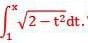Then the real roots of tlie equation x2 — f'(x) = 0 are

Definite Integrals MCQ - 1 - Question 6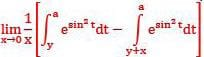is equal to

Detailed Solution for Definite Integrals MCQ - 1 - Question 6

Solution :- limx→0 1/x[∫y→a e^sin2tdt− ∫x+y→a e^sin2tdt]

limx→0  [∫y→a e^sin2tdt− ∫x+y→a e^sin2tdt]/x

Hence it is 0/0 form, Apply L-hospital rule.

lim x-->0  0 - {e^(sin^2a).0 - e^(sin^2(x+y).1}/1

lim x-->0 e^(sin^2(x+y))

= e^(sin^2y)

Definite Integrals MCQ - 1 - Question 7

If f(x) is differentiable and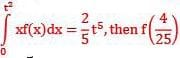equals

Definite Integrals MCQ - 1 - Question 8

If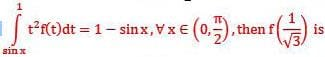Definite Integrals MCQ - 1 - Question 9

The area of the region between the curves y =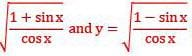and bounded by the lines x = 0 and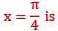Definite Integrals MCQ - 1 - Question 10

Evaluate ∫73sin(t)-2cos(t)dt.

Detailed Solution for Definite Integrals MCQ - 1 - Question 10

Explanation ∫73sin(t)-2cos(t)dt = (cos(t)−2sin(t))73
= (cos(7) – 2sin(7)) – (cos(3) – 2sin(3))
= cos(7) – 2sin(7) – (cos(3) + 2sin(3)

## Topic-wise Tests & Solved Examples for IIT JAM Mathematics

27 docs|150 tests
 Use Code STAYHOME200 and get INR 200 additional OFF Use Coupon Code
Information about Definite Integrals MCQ - 1 Page
In this test you can find the Exam questions for Definite Integrals MCQ - 1 solved & explained in the simplest way possible. Besides giving Questions and answers for Definite Integrals MCQ - 1, EduRev gives you an ample number of Online tests for practice

## Topic-wise Tests & Solved Examples for IIT JAM Mathematics

27 docs|150 tests